# 06 - C Decision Control

In this chapter, we will describe the decision control structure of C language. Decision control structure is responsible for handling situations in programming where alternative execution of instructions is required. In other words, this involves performing a logical test. Result is either true or false and according to that test execution moves further. Let us first have a glance on the available decision structures or conditional control statements are as follows:

• if statement
• if-else statement
• Nested-if statement
• goto statement

## 6.1The if-statement

Single if statement is also known as one-way branching. This is used to execute a statement or set of instructions The body of if statement encapsulates a set of instructions, After performing the logical test if value turns out to be true then the statements inside the body of if is executed, otherwise control moves to the immediate block following the if block. Let us represent the scenario in a diagram so that it is clearer to you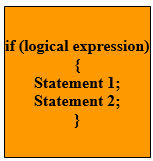Figure - syntax of C if condition

Explanation: In the above diagram,

logical expression is the condition which results in true or false.

Statemet1, Statement 2 constitutes the body of if block

In the above scenario, if the logical expression results true then statement 1 and statement 2 will be executed otherwise the control of execution will move out of this if block.

Example: /* Program to explain the if branching statement*/

```# include<stdio.h>
# include <conio.h>
void main()
{
int a=5;
if (a==5)
{
printf("This statement will appear on screen as logical expression is true\n");
printf("a=%d\n", a);
}
getch();
}
```

Output

This statement will appear on screen as logical expression is true

a=5

Program will look similar to the following snapshot: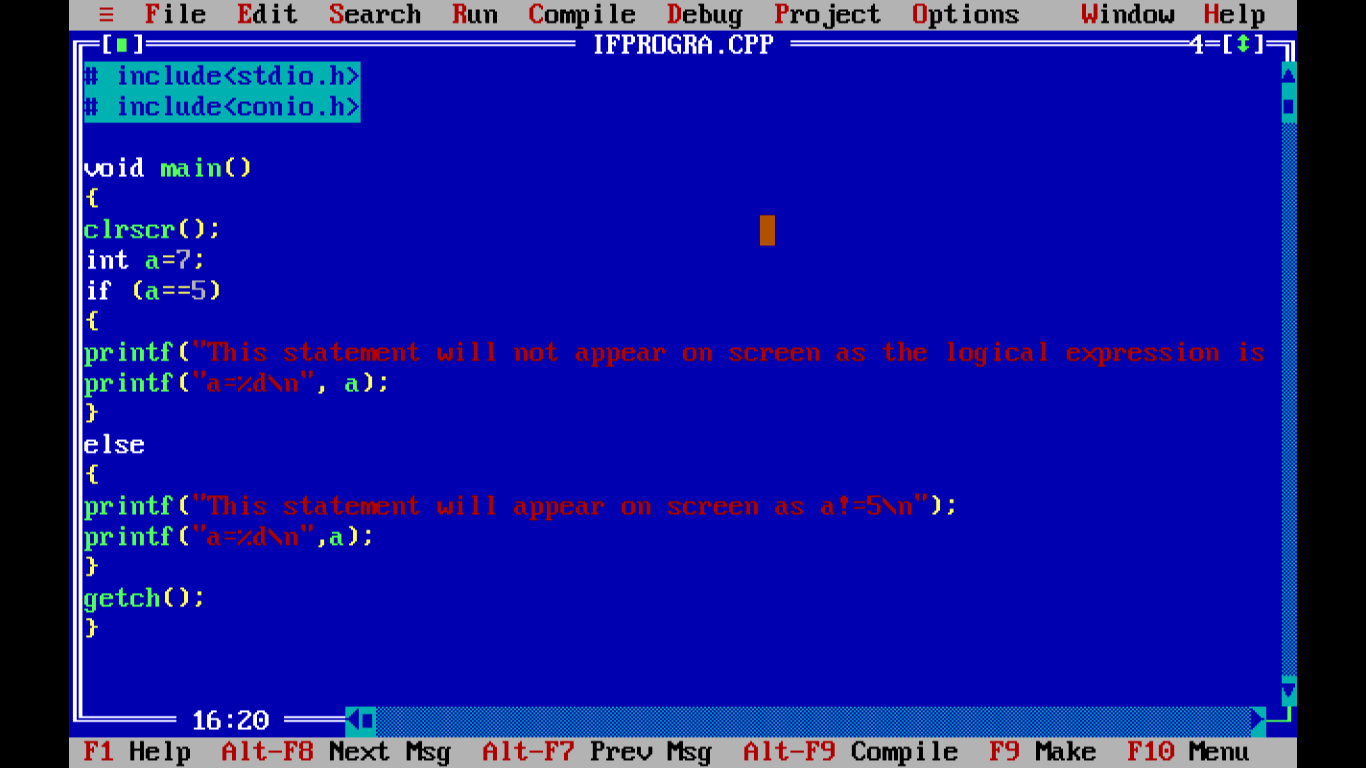Figure – C Program with IF condition in editor

To get the output we need to first compile the program. After compiling the program, execution environment will look similar to following snapshot: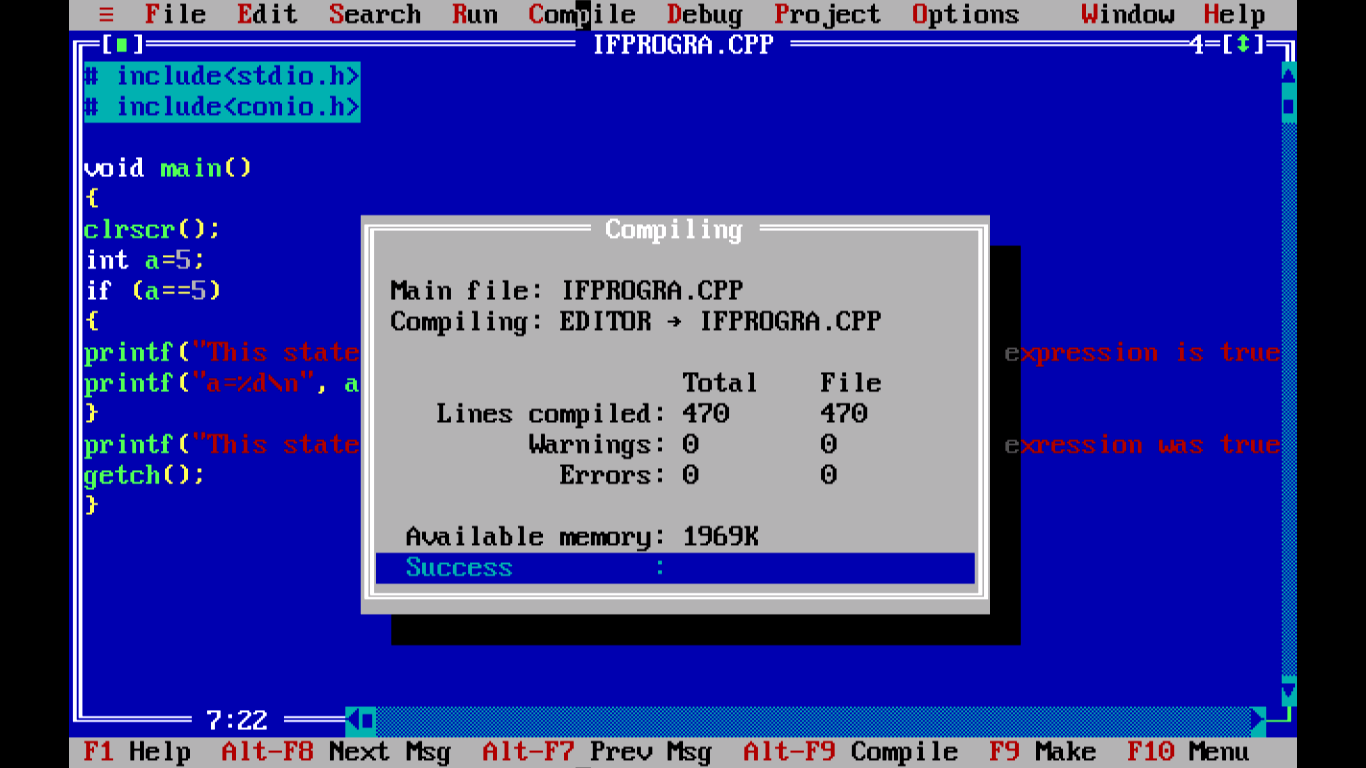Figure - After compiling the C program with IF Condition

After compiling, we need to execute or run the program then the output screen appears. So the out of the program will look similar to the following snapshot: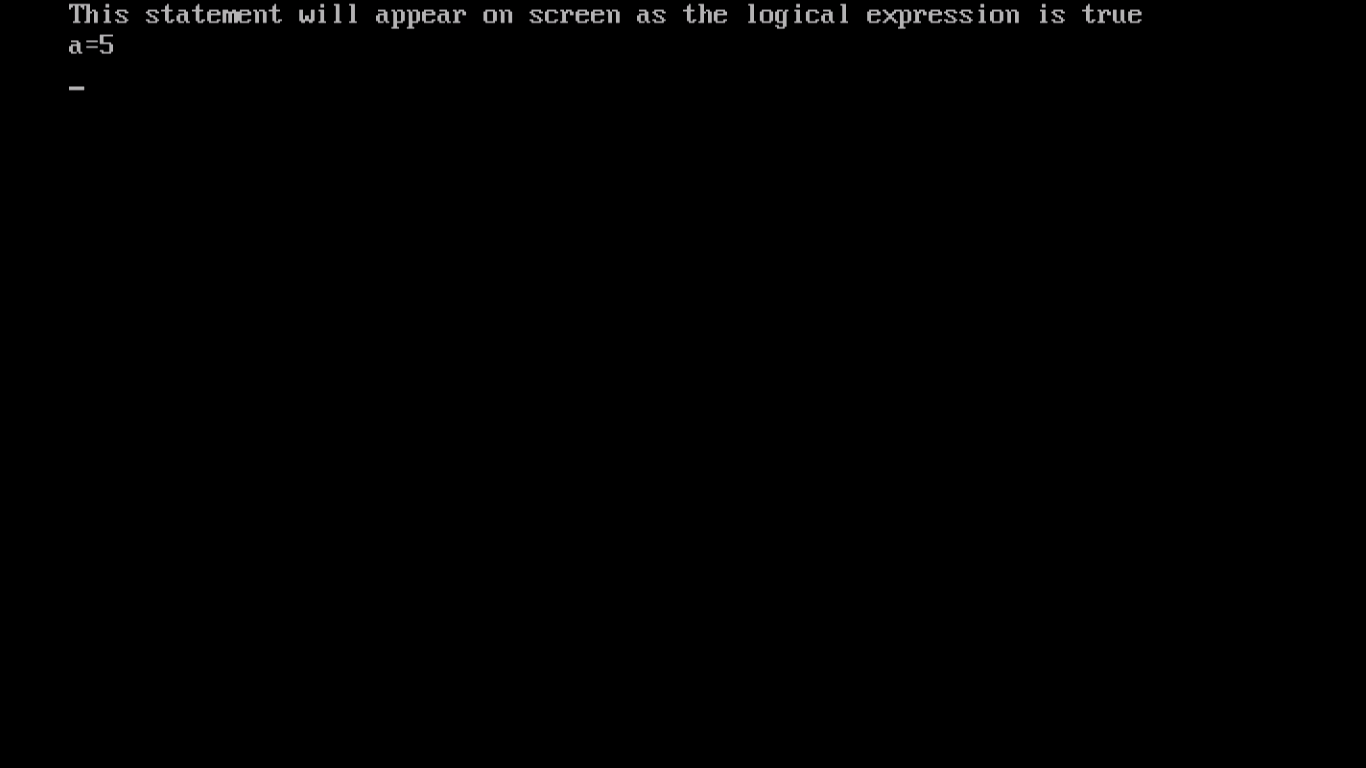Figure - Output of example C program with IF condition

Let us move to next branching statement which the if-else branch or decision control structure.

## 6.1The if-else statement

The if-else block of execution is different from if branching statement although they look similar. The basic difference is that if statement executes only one action. If-else statement is known as two-way branching. In this scenario, logical expression is evaluated to true or false. If the result is true then if block will be executed, if false then else block will be executed. Let us represent the scenario in a diagram so that it is clearer to you.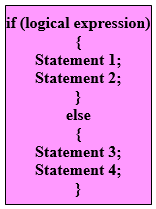Figure – C if-else structure

Explanation: In the above diagram,

logical expression is the condition which results in true or false.

Statemet1, Statement 2 constitutes the body of if block

Statement 3, Statement 4 constitutes the body of else block

In the above diagram, if the logical expression is true then Statement 1 and Statement 2 will be executed which constitutes the if block otherwise Statement 3 and Statement 4 following else block will be executed respectively.

Example: /* Program to illustrate the if-else scenario*/

```# include<stdio.h>
# include <conio.h>
void main()
{
int a=7;
if (a==5)
{
printf("This statement will not appear on screen as logical expression is false\n");
printf("a=%d\n", a);
}
else
{
printf("This statement will appear on screen as a!=5\n");
printf("a=%d\n", a);
}
getch();
}
```

Output

This statement will appear on screen as a!=5

a=7

This program will look similar to the following snapshot:Figure- C Program example with IF-ELSE in editor

After compiling the program will look similar to the following snapshot: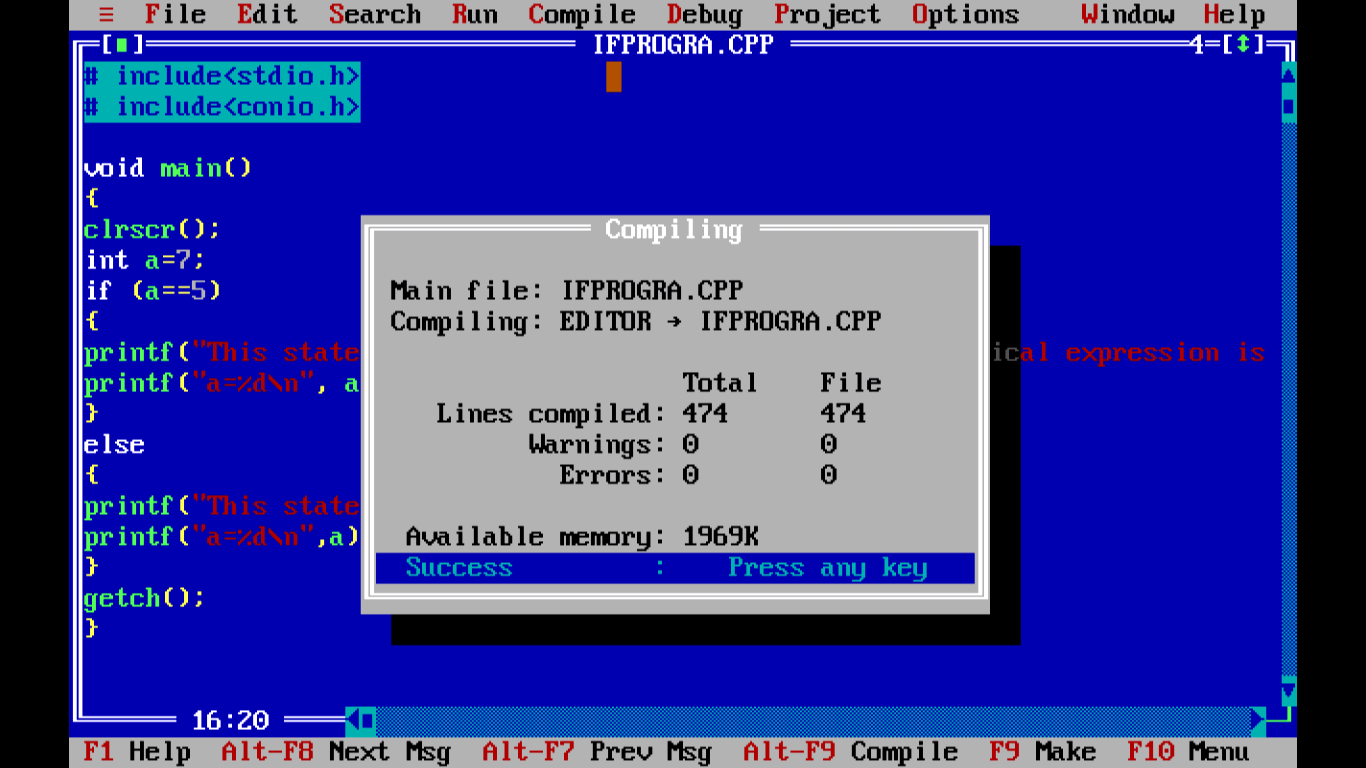Figure -  After compiling the example C program with IF-ELSE

The output of program will look similar to the following snapshot: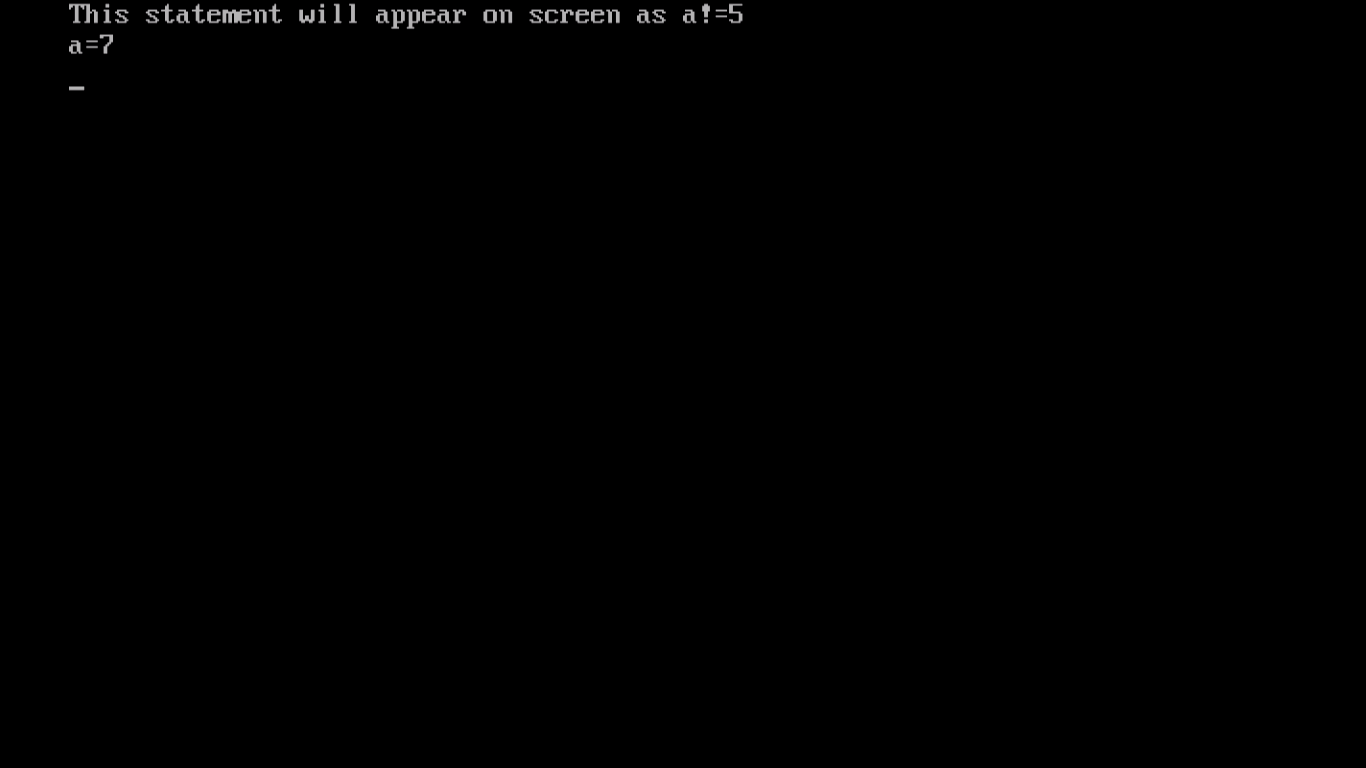Figure - Output of example C program with IF-ELSE

Let us go for the next branching statement or decision control structure.

## 6.3 Nested-if statements

This scenario explains the other scenario. This type of branching is applicable to the situation where more than two alternatives are possible. In this type of scenario, we enclose if block inside another if block. This enclosing of one if statement inside another if statement is called Nested-if statement. Let us represent the scenario in a diagram so that it is clearer to you.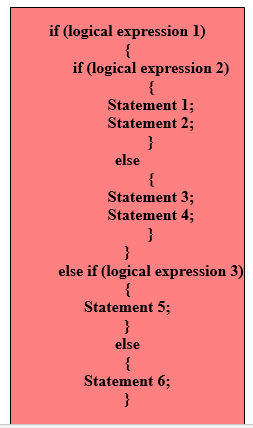​Figure – C Nested-if statement structure

Explanation: In the above example,

logical expression 1, logical expression 2 and logical expression 3 results in true or false

When logical expression 1 is true then control moves to nested if block. Then it checks logical expression 2 is true or false. If it is true, then statement 1 and statement 2 is evaluated. Otherwise, statement 3 and statement 4 will be executed. If logical expression 1 was false then control moves to else if statement. Then logical expression 3 will be evaluated. If it is true, then statement 5 will be executed, control moves to following else statement so statement 6 will be executed. Let us consider an example.

Example: /* Program to demonstrate Nested-if*/

```# include<stdio.h>
# include<conio.h>

void main()
{
int a=7;
if (a<9)
{
if (a==8)
{
printf("This statement will not appear on screen\n");
printf("a=%d\n", a);
}
else
{
printf("This statement will appear on screen\n");
printf("a=%d\n", a);
}
}
else
{
printf("This statement will not appear on screen \n");
printf("a=%d\n", a);
}
getch();
}
```

Output

This statement will appear on screen

a=7

Program will look similar to the following snapshot:Figure – C  Program example with Nested IF in editor

After compiling the program, it will look similar to the following snapshot:Figure -  After compiling the C program with Nested IF

The output of the above program is as follows: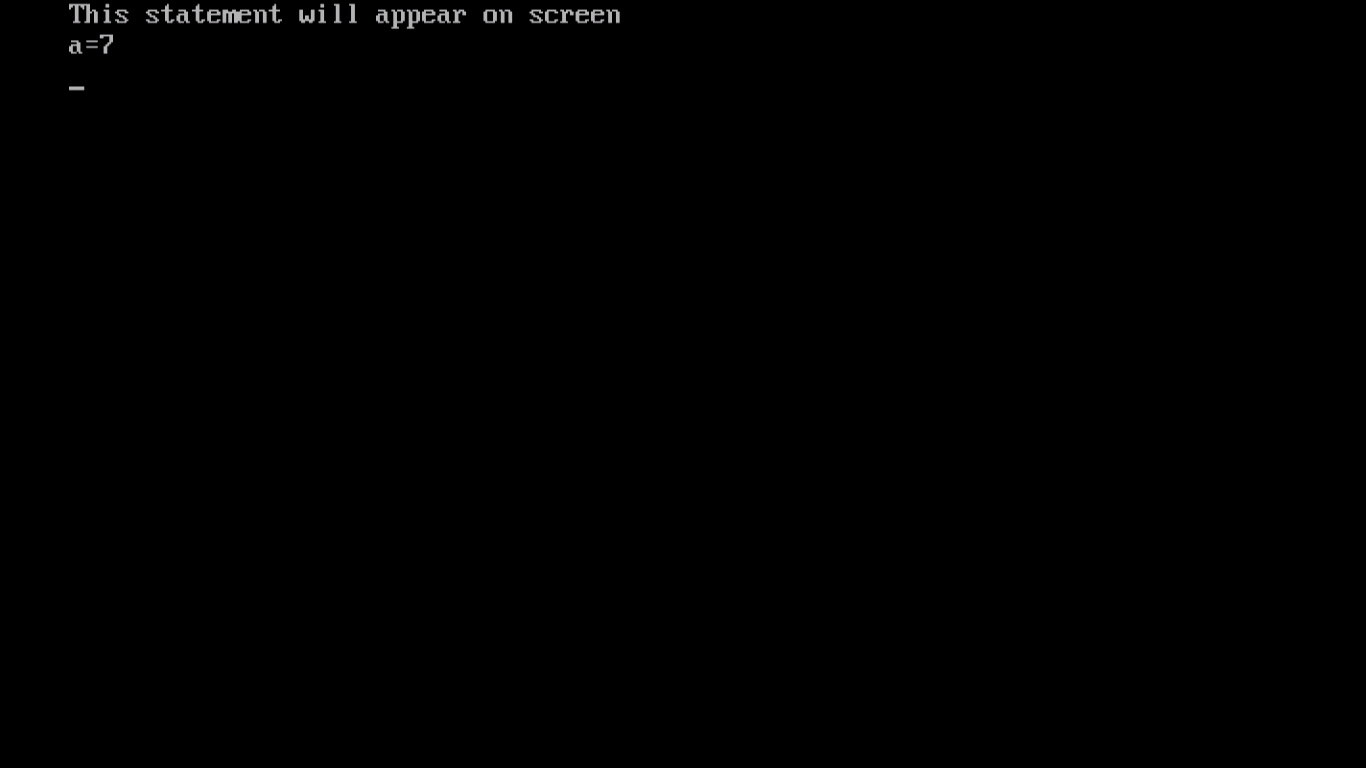Figure -  Output of Example C program with Nested IF

Let us have a look to the last statement which we are going to cover in this chapter.

## 6.4 The goto Statement

This is known as unconditional control statement. This transfers the control of execution to the statement where goto is specified. The goto requires a label for proper execution and label is a symbolic constant.Figure – C goto statement

Let us consider an sample program so that it becomes more clear to you.

Program: /* Program to illustrate goto*/

```# include <stdio.h>
# include <conio.h>
# include <math.h>

void main()
{
clrscr();
int digit=15;
int rem;

START: digit=15 + digit;
rem=digit%5;
if (digit<20)
goto START;
printf ("digit=%d\n", digit);
printf ("rem=%d\n", rem);
getch();
}
```

Output:

digit=30

rem=0

Program looks similar to the following snapshot in the editor: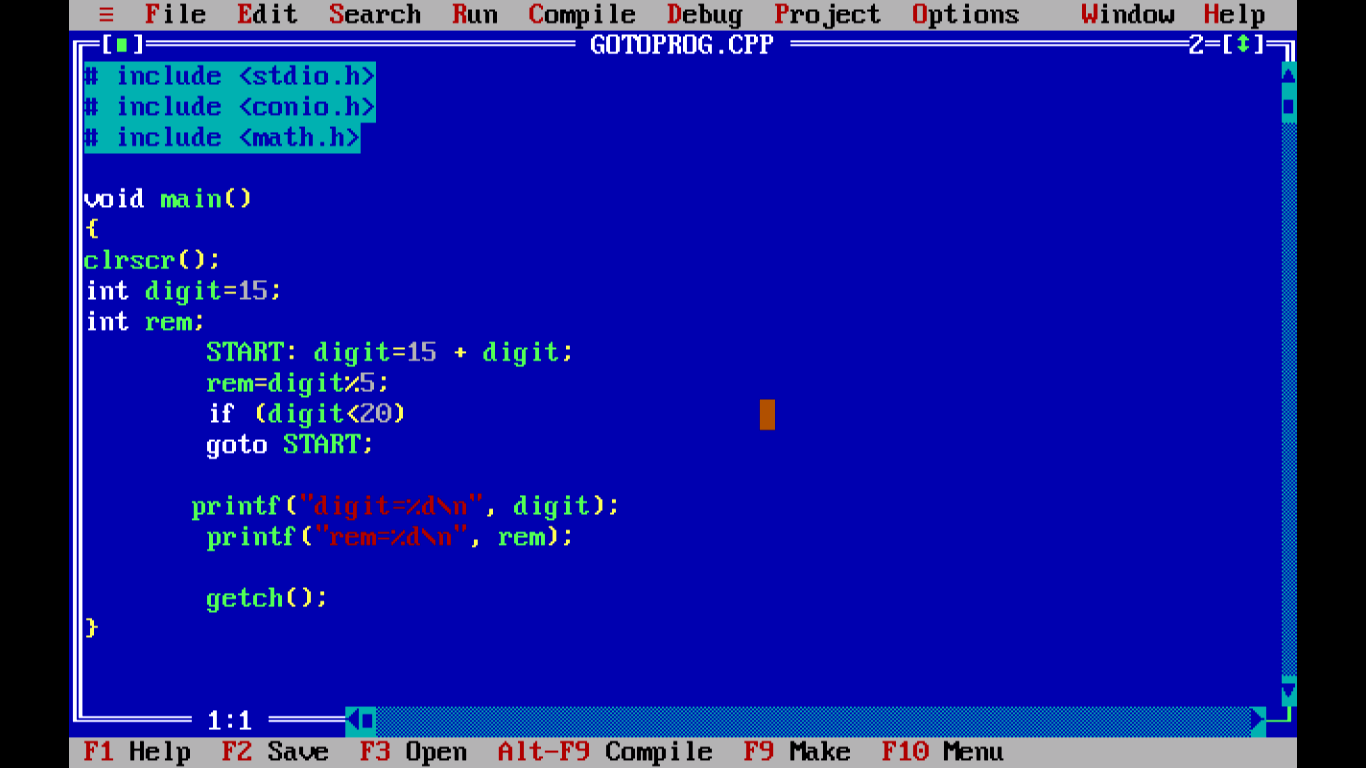Figure - C Program example with GOTO in editor

After compiling the program, compiler will look similar to the following snapshot: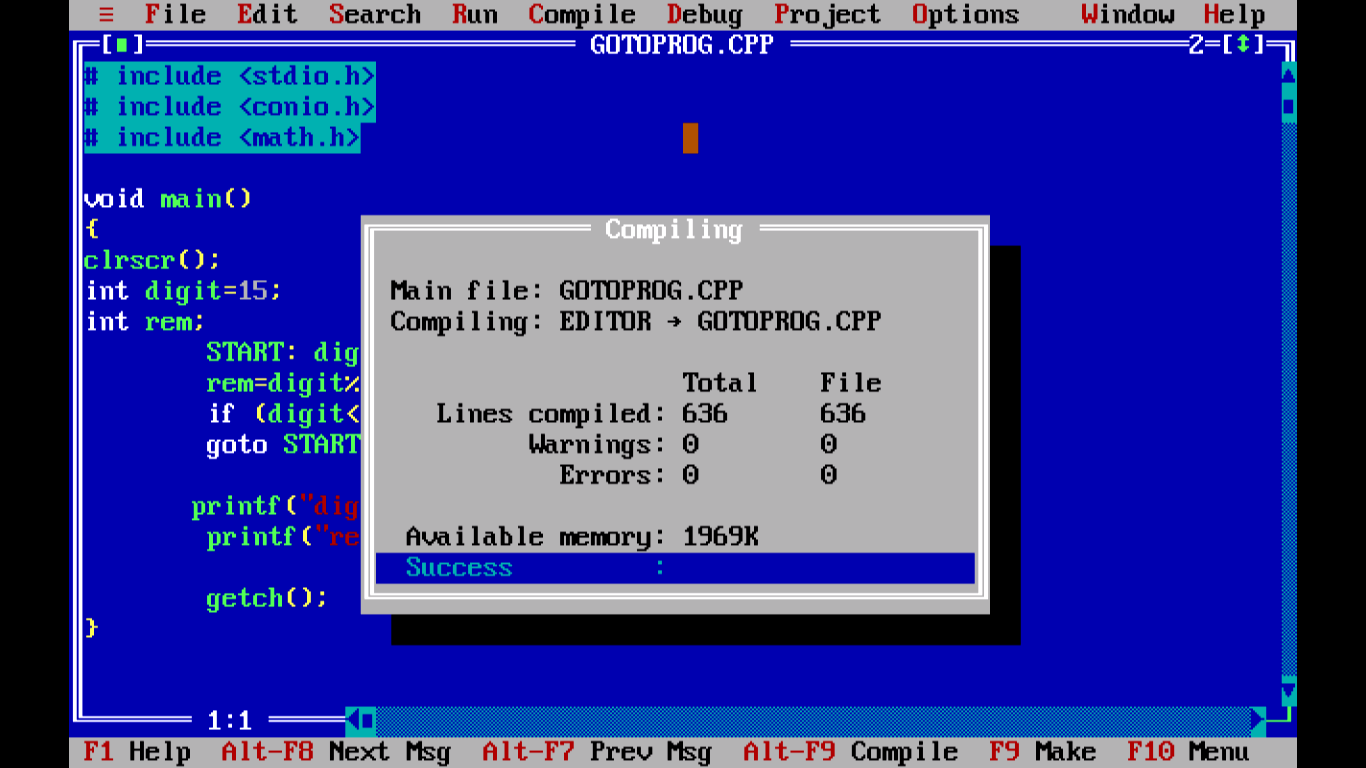Figure - After compiling the example C program with GoTo

Output of the program looks similar to the following snapshot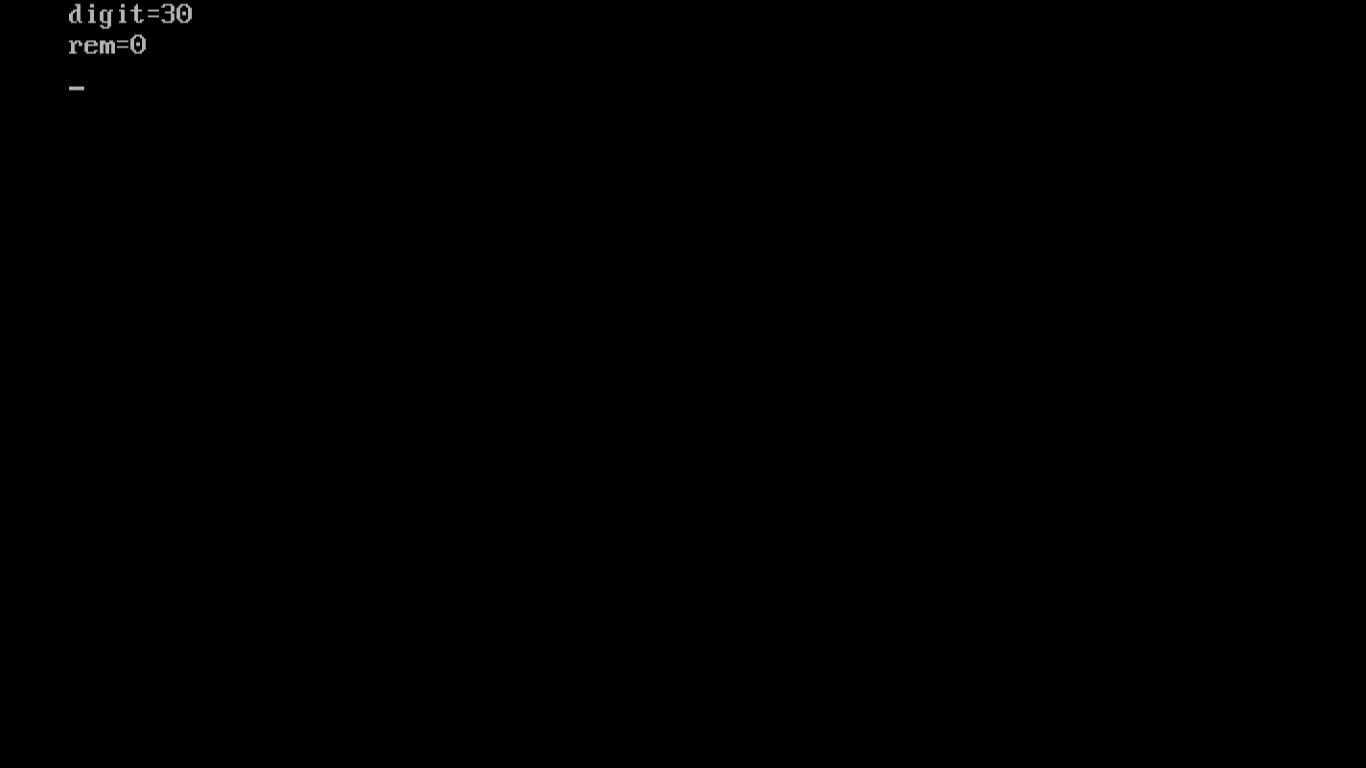Figure - Output of screen example C program with GoTo

With this we conclude this chapter. Next chapter will introduce loop control. Thank you.